# Arithmetic Progressions

 1 Introduction to Arithmetic Progression 2 Arithmetic Progression - Definition 3 Arithmetic Progression - Terminology 4 Common Mistakes 5 Arithmetic Progression - General Term or nth Term 6 Arithmetic Progression - Sum Formula 7 Arithmetic Progressions - Formulas List 8 Solved Examples on Arithmetic Progression 9 Thinking out of the box! 10 Practice Questions on Arithmetic Progression 11 Maths Olympiad Sample Papers 12 Frequently Asked Questions (FAQs)

We at Cuemath believe that Math is a life skill. Our Math Experts focus on the “Why” behind the “What.” Students can explore from a huge range of interactive worksheets, visuals, simulations, practice tests, and more to understand a concept in depth.

Book a FREE trial class today! and experience Cuemath’s LIVE Online Class with your child.

## Introduction

A progression is a sequence of numbers that follow a specific pattern.

In a progression, there is a possibility to derive a formula for the $$n^{th}$$ term.

For example, the sequence $$2, 6, 10, 14, …$$ is a progression because it follows a pattern where each number is obtained by adding $$4$$ to its previous term.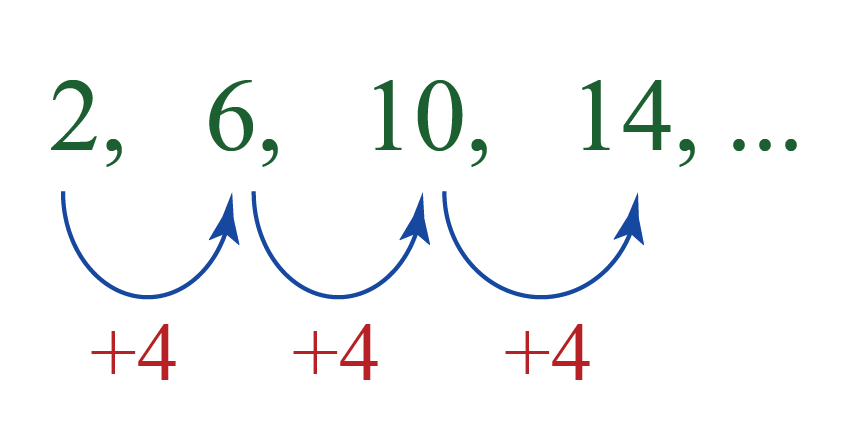In this sequence, $$n^{th}$$ term = $$4n-2$$.

The terms of the sequence can be obtained by substituting $$n=1,2,3,...$$ in the $$n^{th}$$ term.

$\text{When n = 1}, 4n-2 = 4(1)-2 = 4-2=2\\ \text{When n = 2}, 4n-2 = 4(2)-2 = 8-2=6\\ \text{When n = 3}, 4n-2 = 4(3)-2 = 12-2=10\\ .\\ .\\ .$

But how do we find the $$n^{th}$$ term of a given sequence?

## Arithmetic Progression Definition

We can define an arithmetic progression in two ways:

• An arithmetic progression is a sequence where the differences between every two consecutive terms are the same.

• An arithmetic progression is a sequence where each term, except the first term, is obtained by adding a fixed number to its previous term.

For example:

Maria just completed her MCA and she was hired for a job that pays Rs. $$60,000$$ per month with an annual increment of Rs. $$10,000$$ per month. But she expected an initial salary of Rs. $$1,00,000$$ per month.

She wants to know how many years would it take for her to get her desired salary.

Her salary per month would be as follows: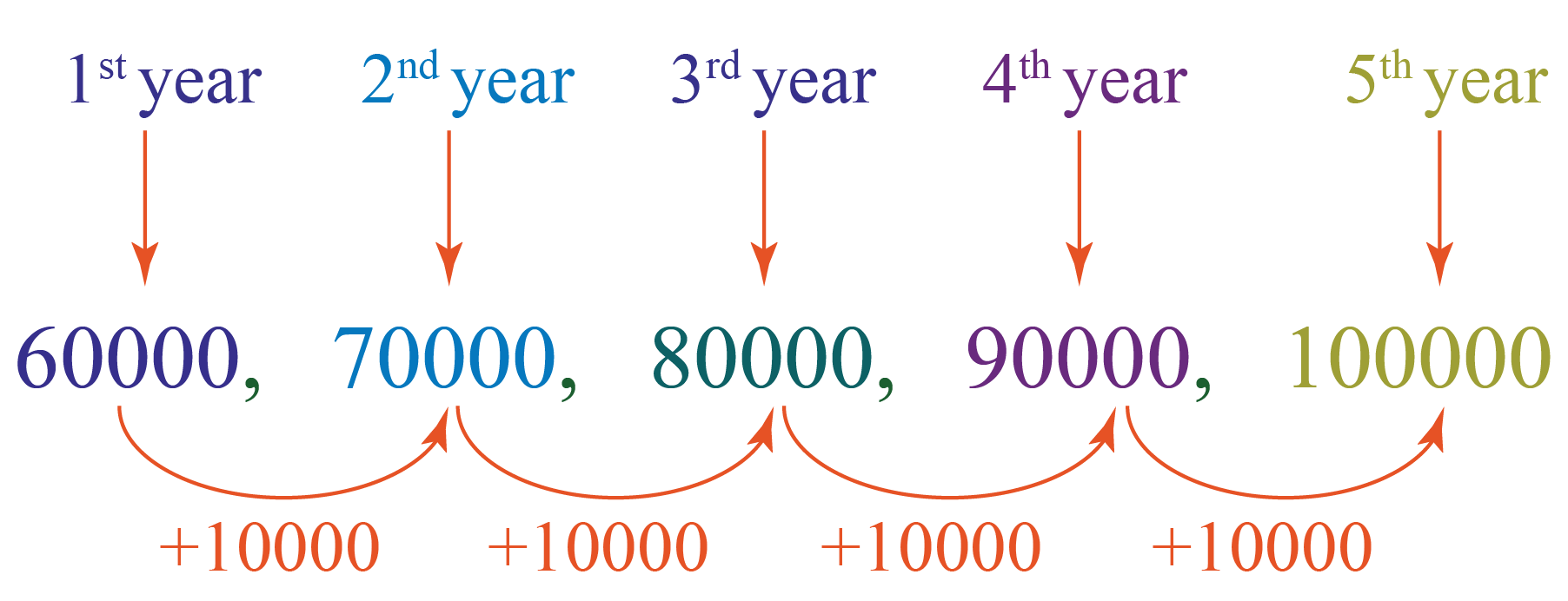She realized that she would earn her desired salary of Rs. $$1,00,000$$ per month in the fifth year of working at this job.

If we observe the above sequence, we can notice that each number, except the first number, is obtained by adding a fixed amount of Rs. $$10000$$ to the previous number.

Thus, by the arithmetic progression definition, the above sequence is an arithmetic progression.

The fixed number, $$10,000$$ is called the “common difference”.

Here is an illustration for you.

We can enter a sequence of numbers here and we can see whether the sequence is an arithmetic progression or not.

## Arithmetic Progression - Terminology

From now on, we will abbreviate arithmetic progression as AP.

Here are some more Arithmetic Progressions examples:

$\begin{array}{l} 6,13,20,27,34, \ldots \\[0.3cm] 91,81,71,61,51, \ldots \\[0.3cm] \pi, 2 \pi, 3 \pi, 4 \pi, 5 \pi, \ldots \\[0.3cm] -\sqrt{3},-2 \sqrt{3},-3 \sqrt{3},-4 \sqrt{3},-5 \sqrt{3}, \ldots \end{array}$

An AP generally is shown as follows:

$a_1, a_2,a_3, \ldots$

It involves the following terminology.

### First Term

As the name suggests, the first term of an AP is the first number of the progression.

It is usually represented by $$a_1$$ (or) $$a$$.

For example, in the sequence $$6,13,20,27,34, \ldots$$, the first term is $$6$$.

i.e., $$a_1=6$$ (or) $$a=6$$.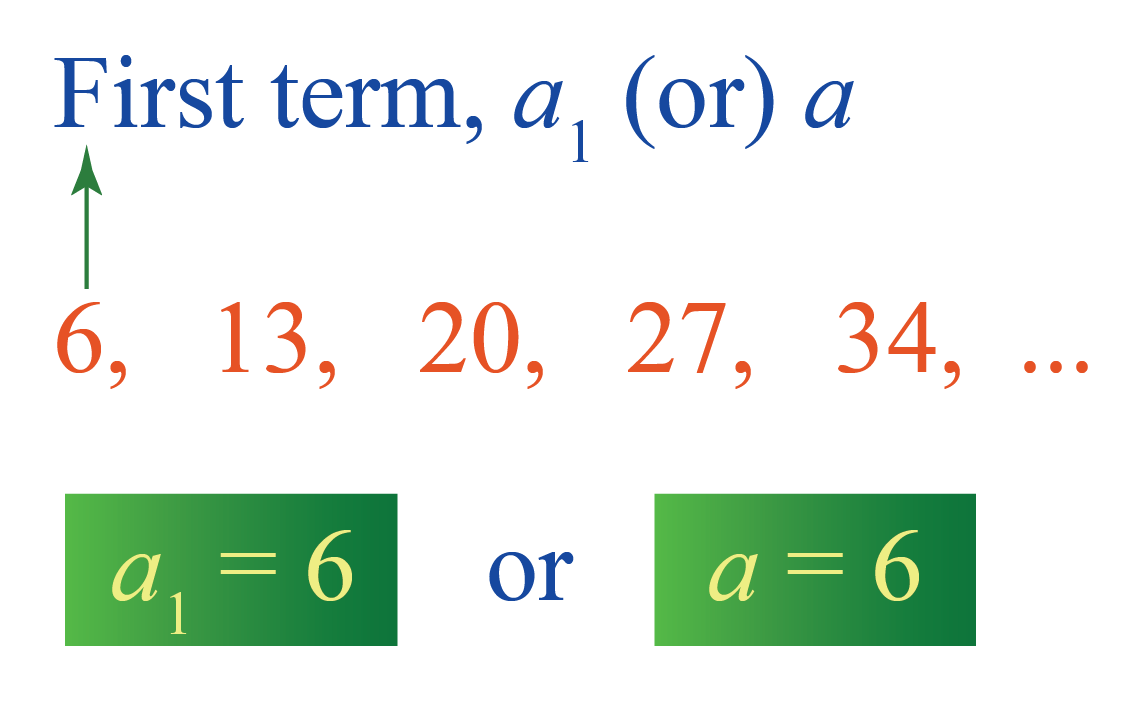CLUEless in Math? Check out how CUEMATH Teachers will explain Arithmetic Progressions to your kid using interactive simulations & worksheets so they never have to memorise anything in Math again!

Explore Cuemath Live, Interactive & Personalised Online Classes to make your kid a Math Expert. Book a FREE trial class today!

### Common Difference

We know that an arithmetic progression is a sequence where each term, except the first term, is obtained by adding a fixed number to its previous term.

Here, the “fixed number” is called the “common difference” and is denoted by $$d$$.

i.e., if the first term is $$a_1$$, then:

• the second term is $$a_1+d$$.
• the third term is $$a_1+d+d = a_1+2d$$.
• the fourth term is $$a_1+2d+d= a_1+3d$$ and so on.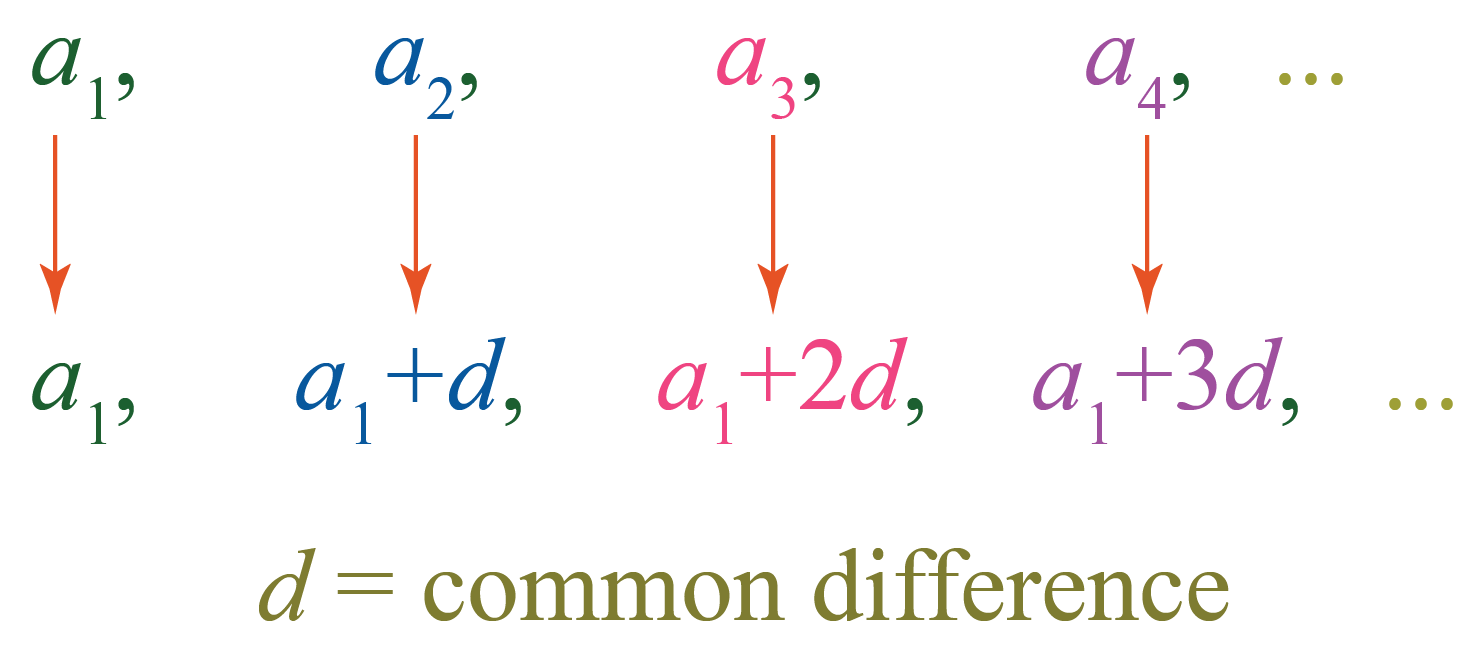For example, in the sequence $$6,13,20,27,34, \ldots$$, each term, except the first term, is obtained by adding $$7$$ to its previous term.

Thus, the common difference is, $$d=7$$.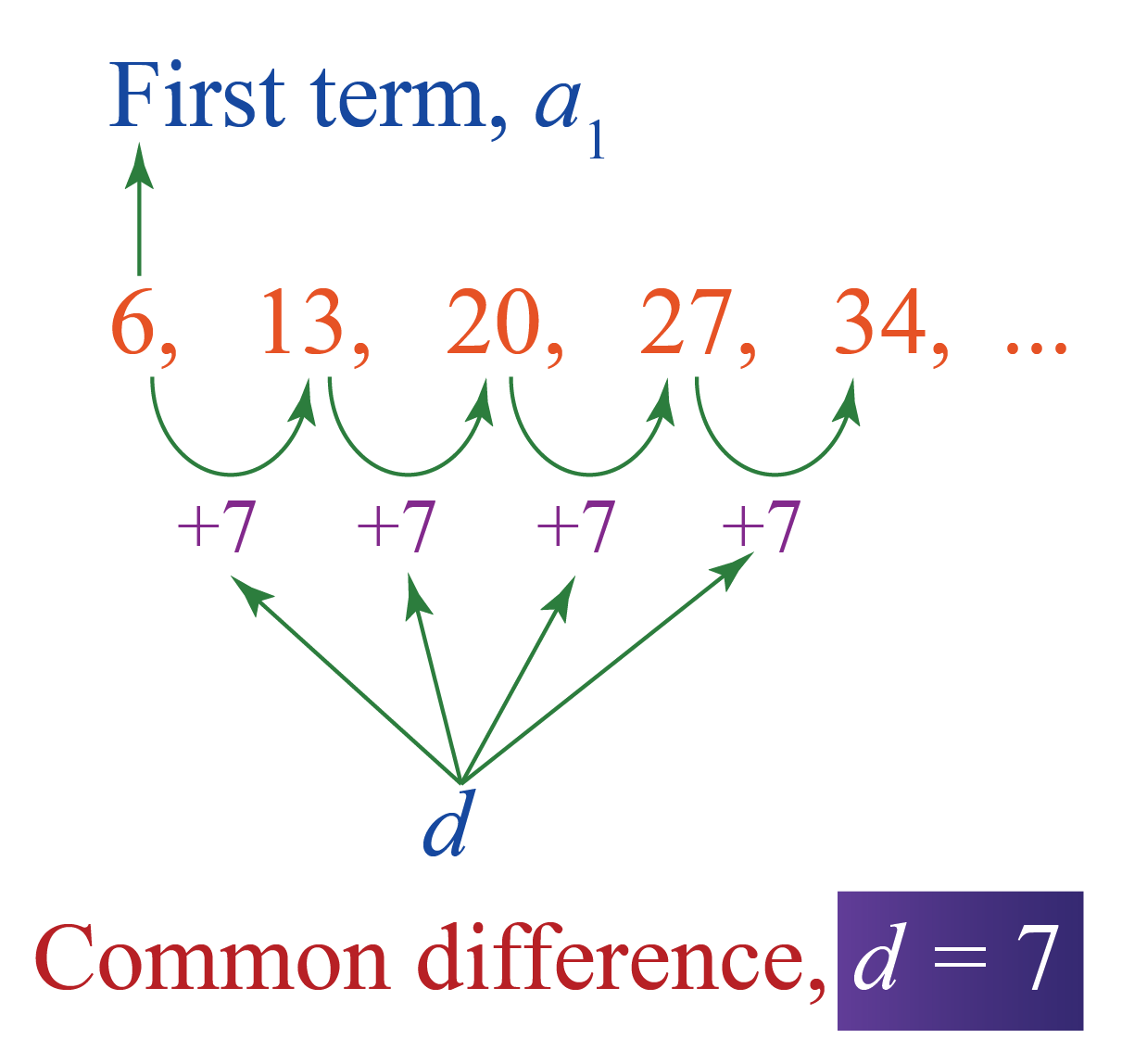In general, the common difference is the difference between every two successive terms of an AP.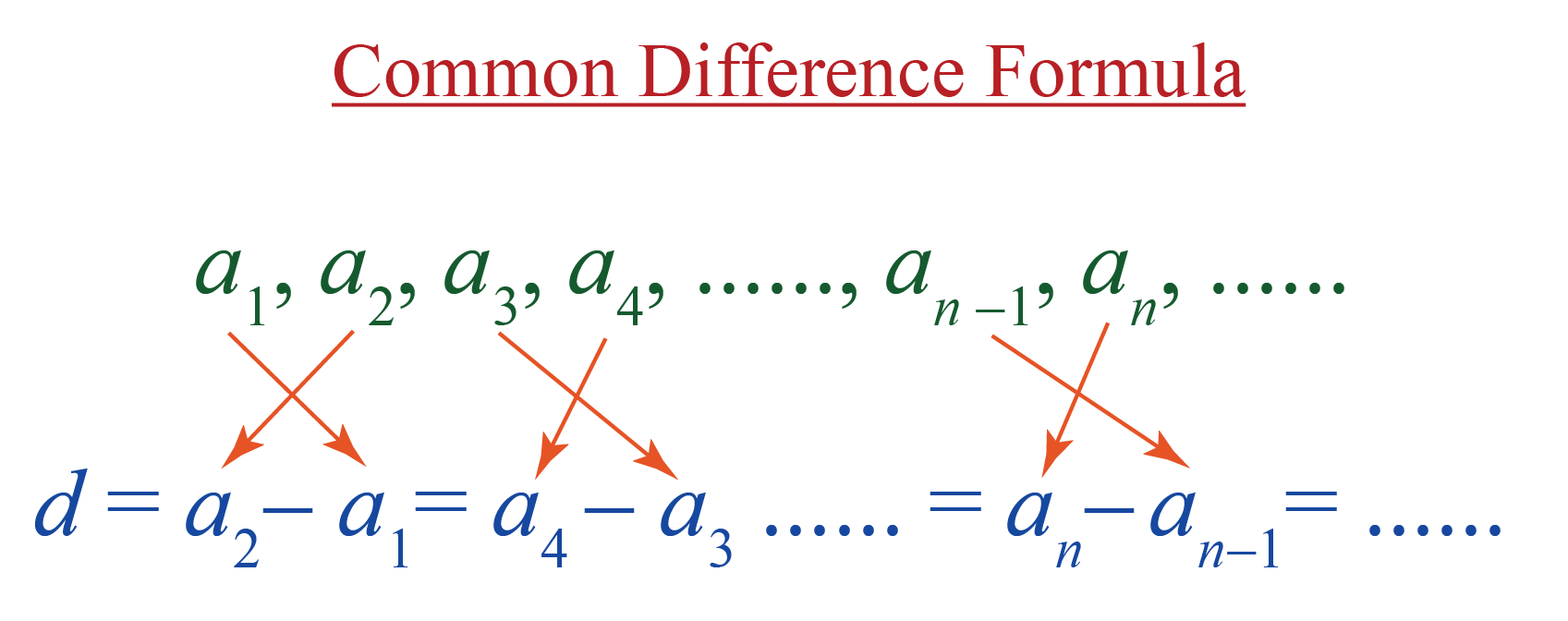Thus, the formula for calculating the common difference of an AP is:

 $$d=a_n-a_{n-1}$$Common Mistakes
1. Mistake: The common difference is always positive.

Fact: The common difference doesn't need to be positive always.

For example, in the sequence, $$16, 8, 0, -8, -16,....$$, the common difference is,

$$d$$ = 8 - 16 = 0 - 8 = -8 - 0 = -16 - (-8) =... = -8

Here the common difference is negative.

2. Mistake: The common difference an AP is the difference between two consecutive terms of the AP in any order.

Fact: The common difference of an AP is the difference between two consecutive terms of the AP taken in this order.

$\text{Term - Previous term}$

## Arithmetic Progression - General Term or nth Term

The general term (or) $$n^{th}$$ term of an arithmetic progression whose first term is $$a$$ and common difference is $$d$$ is found by the formula:

 $$a_n=a+(n-1)d$$

For example,

To find the general term (or) $$n^{th}$$ term of the sequence $$6,13,20,27,34, \ldots$$, we substitute the first term, $$a_1=6$$ and the common difference, $$d=7$$ in the above formula.

Then we get,

\begin{align} a_n&=a+(n-1)d\\ a_n &= 6+(n-1)7\\ a_n&= 6+7n-7 \\ a_n&= 7n -1 \end{align}

Thus, the general term (or) $$n^{th}$$ term of the above sequence is:

$a_n = 7n-1$

But what is the use of finding the general term of an AP?

We know that to find a term, we can add $$d$$ to its previous term.

For example, if we have to find the $$6^{th}$$ term of $$6,13,20,27,34, \ldots$$, we can just add $$d=7$$ to the $$5^{th}$$ term which is $$34$$.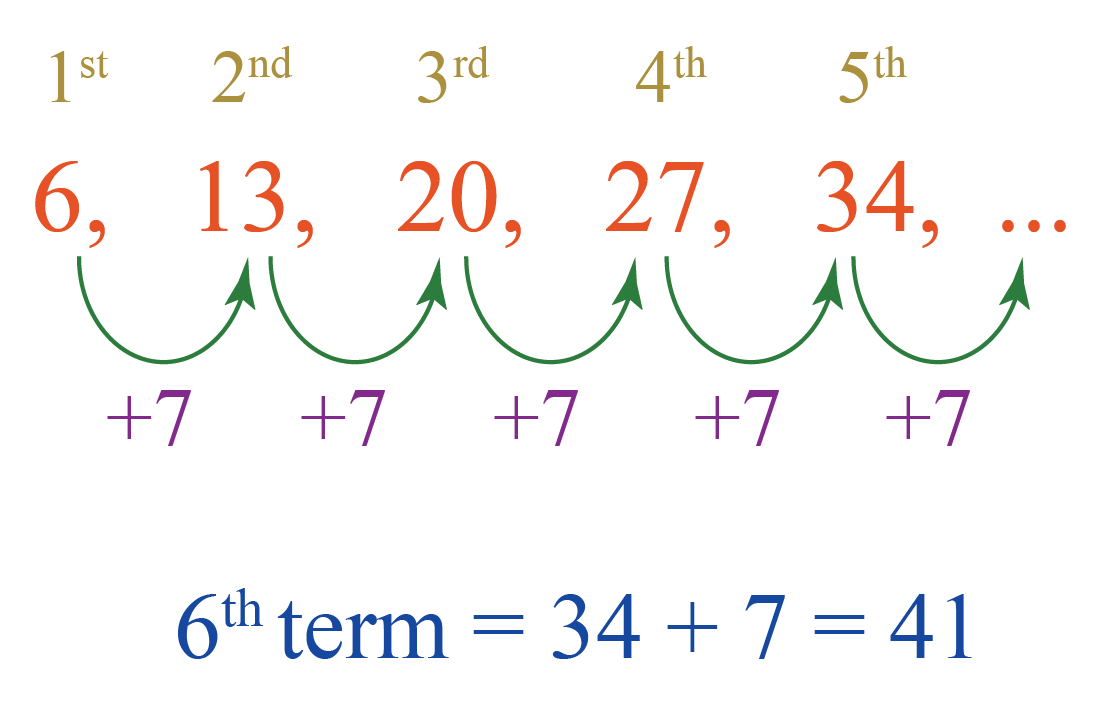$6^{th} \text{ term} = 5^{th} \text{ term}+ 7 = 34+7 = 41$

But what if we have to find the $$102^{nd}$$ term?

Isn’t it difficult to calculate it manually?

In this case, we can just substitute $$n=102$$ (and also $$a=6$$ and $$d=7$$ in the formula of $$n^{th}$$ term of an AP.

Then we get:

\begin{align} a_n&=a+(n-1)d\\ a_{102} &= 6+(102-1)7\\ a_{102}&= 6+(101)7 \\ a_{102}&= 713 \end{align}

Therefore, the $$102^{nd}$$ term of the above sequence is $$713$$.

Thus, the general term (or) $$n^{th}$$ term of an AP is used to find any term of the AP without finding its previous term.

The following table shows some arithmetic progression examples and the first term, the common difference and the general term in each case.

Arithmetic Progression First Term Common Difference

General term

$$n^{th}$$ term

AP $$a$$ $$d$$ $$a_n=a+(n-1)d$$
$$91,81,71,61,51, \ldots$$ $$91$$ $$-10$$ \begin{align} -10n+101 \end{align}
$$\pi, 2 \pi, 3 \pi, 4 \pi, 5 \pi, \ldots$$ $$\pi$$ $$\pi$$
\begin{align} \pi n \end{align}
$$-\sqrt{3},-2 \sqrt{3},-3 \sqrt{3},-4 \sqrt{3}, \ldots$$ $$-\sqrt{3}$$ $$-\sqrt{3}$$
\begin{align} -\sqrt{3} n \end{align}

## Arithmetic Progression - Sum Formula

Consider an arithmetic progression (AP) whose first term is $$a_1$$ (or) $$a$$ and the common difference is $$d$$.

• The sum of first $$n$$ terms of an arithmetic progression when the $$n^{th}$$ term is NOT known is:
 $S_n=\frac{n}{2}[2 a+(n-1) d]$
• The sum of first $$n$$ terms of an arithmetic progression when the $$n^{th}$$ term, $$a_n$$ is known is:
 $S_n=\frac{n}{2}[a_1+a_n]$

Example:

Mr. Karan earns Rs. $$4,00,000$$ per annum and his salary increases by Rs. $$50,000$$ per annum. Then how much does he earn at the end of first  $$3$$ years?

Solution:

The amount earned by Mr. Karan for the first year is, $$a=4,00,000$$.

The increment per annum is, $$d=50,000$$.

We have to calculate his earnings in the $$3$$ years. So $$n=3$$.

Substituting these values in the arithmetic progression sum formula,

\begin{align} S_n&=\frac{n}{2}[2 a+(n-1) d] \\[0.3cm] S_3 &= \frac{3}{2}(2(400000)+(3-1)(50000)) \\[0.3cm] &= \frac{3}{2} (800000+ 100000)\\[0.3cm] &= \frac{3}{2} (900000)\\[0.3cm] &= 1350000 \end{align}

 $$\therefore \text{He earned Rs. 13,50,000 in 3 years}$$

We can get the same answer by general thinking also as follows:

The annual amount earned by Mr. Karan in the first three years is as follows.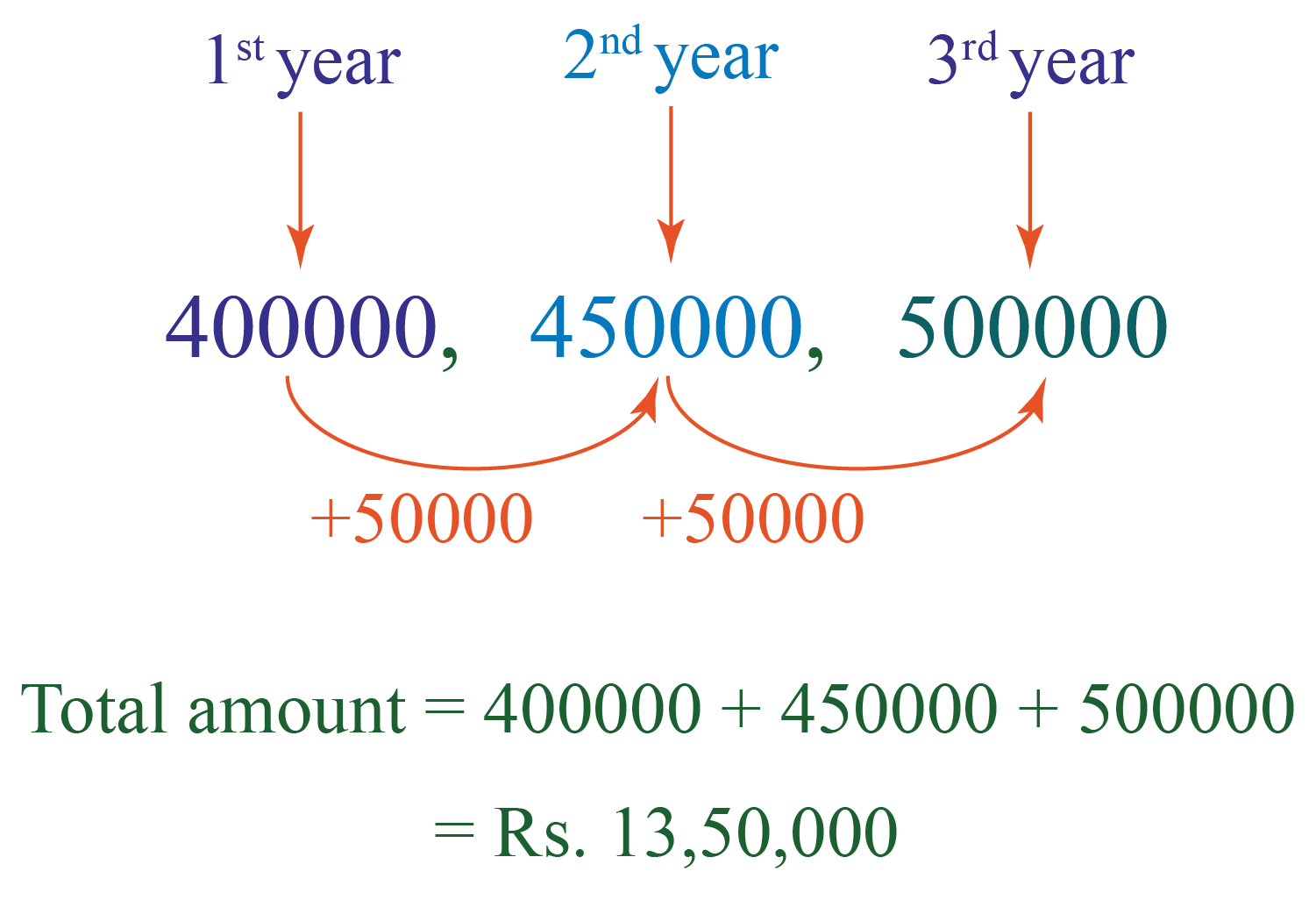This could be calculated manually as $$n$$ is a smaller value.

But the above formulas are useful when $$n$$ is a larger value.

Help your child score higher with Cuemath’s proprietary FREE Diagnostic Test. Get access to detailed reports, customised learning plans and a FREE counselling session. Attempt the test now.

## Arithmetic Progressions - Formulas List

The formulas related to arithmetic progression for class 10th are:

 Common difference of an AP $$d=a_n−a_{n−1}$$ $$n^{th}$$ term of an AP $$a_n=a+(n-1)d$$ Sum of $$n$$ terms of an AP \begin{align} S_n &=\frac{n}{2} (2a+(n-1)d) \end{align}

## Solved Examples

Here are some arithmetic progression problems/arithmetic progression sums.

 Example 1

Find the general term of the AP $$-3, -\dfrac{1}{2},2…$$.

Solution:

The given sequence is:

$-3, -\frac{1}{2},2…$

Here, the first term is:

$a=-3$

The common difference is,

$d = -\frac{1}{2} -(-3) = -\frac{1}{2}+3 = \frac{5}{2}$

The general term of an AP is calculated by the formula:

\begin{align} a_n &= a+(n-1)d \\[0.3cm] a_n &= -3 +(n-1) \frac{5}{2}\\[0.3cm] &= -3+\frac{5}{2} n - \frac{5}{2}\\[0.3cm] &= \frac{5}{2} n -\frac{11}{2} \end{align}

Therefore, the general term of the given AP is:

 $$a_n = \frac{5}{2} n -\frac{11}{2}$$
 Example 2

Which term of the AP $$3, 8, 13, 18,...$$ is $$78$$?

Solution:

The given sequence is:

$3,8,13,18,...$

Here, the first term is,

$a=3$

The common difference is,

$d = 8-3= 13-8=...=5$

Let us assume that the $$n^{th}$$ term is,

$a_n=78$

Substitute all these values in the general term of an AP:

\begin{align} a_n &= a+(n-1)d \\[0.3cm] 78 &= 3+(n-1)5\\[0.3cm] 78&=3+5n-5\\[0.3cm] 78&= 5n -2 \\[0.3cm] 80 &=5n\\[0.3cm] 16&=n \end{align}

 $$\therefore 78 \text{ is the } 16^{th} \text{ term}$$
 Example 3

Find the sum of the AP $$2, 5, 8, …$$ upto $$10$$ terms.

Solution:

The given sequence is:

$2, 5, 8, …$

Here, the first term is,

$a=2$

The common difference is,

$d = 5-2=8-5=...=3$

We have to find the sum of $$10$$ terms. So

$n=10$

Substitute all these values in the an arithmetic progression sum formula:

\begin{align} S_n&= \frac{n}{2} (2a+(n-1)d)\\[0.3cm] S_{10} &= \frac{10}{2}(2(2)+(10-1)3) \\[0.3cm] &= 5(4+9(3))\\[0.3cm] &= 5(4+27)\\[0.3cm] &= 5(31)\\[0.3cm] &= 155 \end{align}

 $$\text{Sum of 10 terms of given AP} = 155$$Think Tank
1. What is the general term of the AP $$16,13,10, \ldots$$?
2. Check whether $$301$$ is a term for the AP $$5,11,17,23, \ldots$$
3. What is the sum of first $$50$$ multiples of $$3$$?
4. How many multiples of $$4$$ lie between $$8$$ and $$200$$?## Practice Questions

IMO (International Maths Olympiad) is a competitive exam in Mathematics conducted annually for school students. It encourages children to develop their math solving skills from a competition perspective.

## 1. What is arithmetic progression with example?

We can define an arithmetic progression in two ways.

An arithmetic progression is a sequence where the differences between every two consecutive terms are the same.

An arithmetic progression is a sequence where each term, except the first term, is obtained by adding a fixed number to its previous term.

Here are some more Arithmetic Progression examples:

$\begin{array}{l} 6,13,20,27,34, \ldots \\[0.3cm] 91,81,71,61,51, \ldots \\[0.3cm] \pi, 2 \pi, 3 \pi, 4 \pi, 5 \pi, \ldots \\[0.3cm] -\sqrt{3},-2 \sqrt{3},-3 \sqrt{3},-4 \sqrt{3},-5 \sqrt{3}, \ldots \end{array}$

We can also find some arithmetic progression questions under the "Solved Examples" and "Think Tank" sections of this page.

## 2. What are the types of arithmetic progression?

An arithmetic progression is a sequence where the differences between every two consecutive terms are the same.

Arithmetic progression is not classified into any other types. However, there are three types of progressions. They are:

• Arithmetic progressions
• Geometric progressions
• Harmonic progressions

## 3. What is the formula for the sum of arithmetic progression?

The formulas related to arithmetic progression for class 10th are:

 Sum of $$n$$ terms of an AP \begin{align} S_n &=\frac{n}{2} (2a+(n-1)d) \end{align}

 Sum of $$n$$ terms of an AP \begin{align} S_n &=\frac{n}{2} (2a+(n-1)d) \end{align} Sum of $$n$$ terms of an AP \begin{align} S_n &=\frac{n}{2} (a_1+a_n) \end{align}

We can see the arithmetic progression problems/arithmetic progression sums as well in this article in the Practice Questions section.

## 4. Where is arithmetic progression used?

An arithmetic progression is used when we come up with a sequence of numbers where every two consecutive terms has the same difference.

You can refer to the “Arithmetic Progression Definition” section of this page for a real-life example.

More Important Topics
Numbers
Algebra
Geometry
Measurement
Money
Data
Trigonometry
Calculus